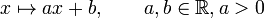# Characteristic not implies powering-invariant in solvable group

This article gives the statement and possibly, proof, of a non-implication relation between two subgroup properties, when the big group is a solvable group. That is, it states that in a solvable group, every subgroup satisfying the first subgroup property (i.e., characteristic subgroup) need not satisfy the second subgroup property (i.e., powering-invariant subgroup)
View all subgroup property non-implications | View all subgroup property implications

## Statement

It is possible to construct a solvable group$G$ and a characteristic subgroup$H$ of$G$ such that$H$ is not a powering-invariant subgroup of$G$.

In fact, we can choose$G$ to be powered for any set of primes and$H$ to be powered over any choice of subset of the set of primes (and not over the others).

## Proof

### Example of a rationally powered group and a characteristic subgroup that is not powered for any prime

Further information: GAPlus(1,R)

Suppose$G$ is the group$GA^+(1,\R)$, given explicitly as the group of linear maps with positive coefficient from$\R$ to itself under composition. Explicitly, the elements of$G$ are maps of the form:$x \mapsto ax + b, \qquad a,b \in \R, a > 0$

We can think of$G$ as the semidirect product$\R \rtimes (\R^\ast)^+$, i.e., the semidirect product of the reals under addition by the action of the positive reals under multiplication with their natural action by multiplication.

Suppose$H$ is the subgroup of$G$ given by the semidirect product$\R \rtimes (\mathbb{Q}^\ast)^+$. Explicitly,$H$ is the subgroup comprising the maps of the form:$x \mapsto ax + b, a \in \mathbb{Q}, b \in \R, a > 0$

Then, we note that:

•$G$ is rationally powered: See GAPlus(1,R) is rationally powered
•$H$ is not powered for any prime: For instance, the map$x \mapsto 2x$ is in$H$. For any prime$p$, its unique$p^{th}$ root in$G$ is$x \mapsto 2^{1/p}x$. But since$2^{1/p} \notin \mathbb{Q}$, this element is not in$H$.
•$H$ is characteristic in$G$: First, note that the translation subgroup$x \mapsto x + b$ is precisely the derived subgroup$[G,G]$, hence it is characteristic. This subgroup is the base of the semidirect product and is isomorphic to the additive group of$\R$, hence is rationally powered. Now, note that$H$ is precisely the subgroup of$G$ of elements such that the automorphism of$[G,G]$ induced by its action by conjugation is a rational multiplication. Note that whether an automorphism is a rational multiplication is a purely group-theoretic condition, so the above specifies$H$ uniquely in an automorphism-invariant manner.

### Example for suitably chosen sets of primes

Consider two prime sets$\pi_1$ and$\pi_2$ with$\pi_2 \subset \pi_1$ (as a proper subset). Define the following two subsets of$\R$:

•$T_1$ is the subset of the set of positive reals comprising those numbers$x$ for which there is a natural number$n$ with all prime factors in$\pi_1$ for which$x^n \in (\mathbb{Q}^\ast)^+$.
•$T_2$ is the subset of the set of positive reals comprising those numbers$x$ for which there is a natural number$n$ with all prime factors in$\pi_2$ for which$x^n \in (\mathbb{Q}^\ast)^+$.

Note that$T_2 \subset T_1$ because$\pi_2 \subset \pi_1$.

Now define$G$ and$H$ as the following groups of linear maps from$\R$ to$\R$:$G = \{ x \mapsto ax + b, a \in T_1, b \in \R \}$$H = \{ x \mapsto ax + b, a \in T_2, b \in \R \}$

Then, we can see that:

•$G$ is powered precisely over the primes in$\pi_1$ and over no other primes
•$H$ is powered precisely over the primes in$\pi_2$ and over no other primes
•$H$ is a characteristic subgroup of$G$